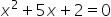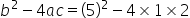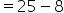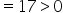Mathematics
Easy

Question

# How many solutions are there for the equationusing the discriminant?

## 0    1    2    3Hint:

## The correct answer is: 2

### Step 1 of 1:We have given a quadratic equationThe discriminant is greater than zero, so it has two real solutions.

The discriminant is the part of the quadratic formula found within the square root.

### Related Questions to study#### With Turito Foundation.#### Get an Expert Advice From Turito.